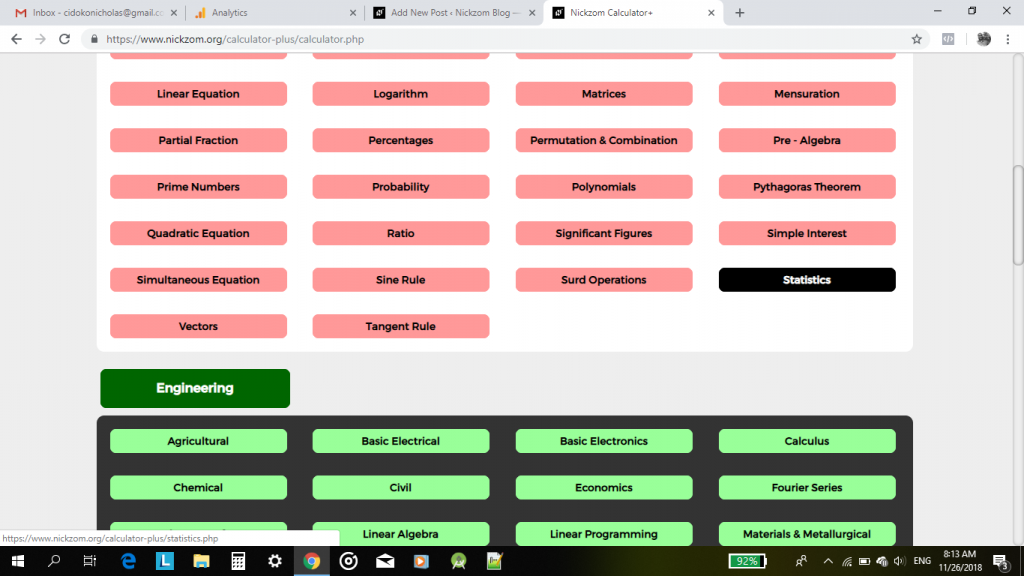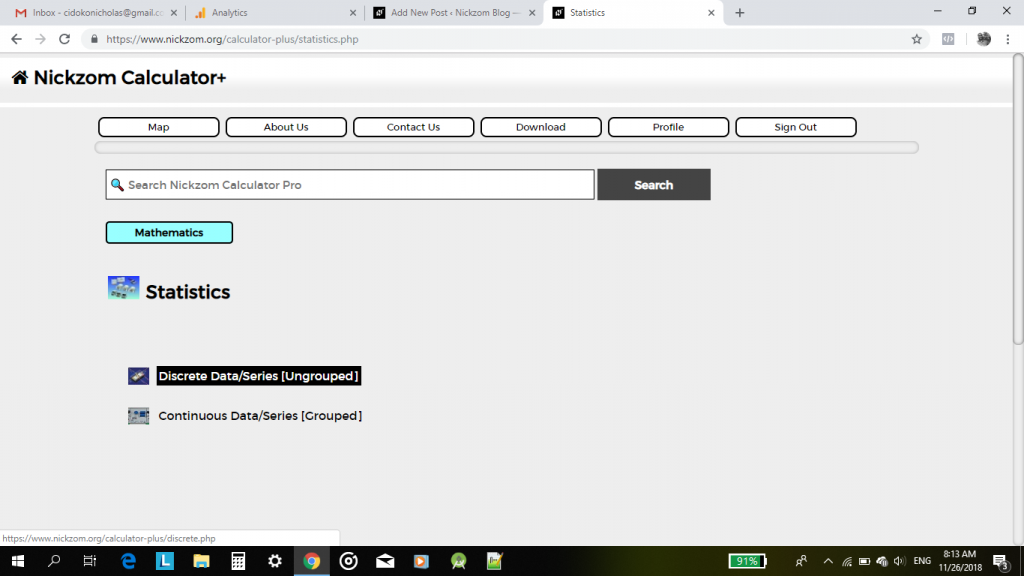# Nickzom Calculator Calculates the Mean for Discrete and Continuous Data with Frequency under Statistics

Nickzom Calculator is capable of calculating a lot of problems on statistics and one of them is mean under measures of central tendency.

To this is very simple.

To access this functionalities in Nickzom Calculator, you need to purchase our professional version – Nickzom Calculator+ (The Calculator Encyclopedia). You can access this software via any of these channels:

Straight up go to Calculator MapClick on StatisticsBelow, is a video displaying Nickzom Calculator solving the mathematical mean  of discrete and continuous data with and without frequencies.

According to Wikipedia,

For a data set, the arithmetic mean, also called the mathematical expectation or average, is the central value of a discrete set of numbers: specifically, the sum of the values divided by the number of values. The arithmetic mean of a set of numbers x1x2, …, xn is typically pronounced “x bar”. If the data set were based on a series of observations obtained by sampling from a statistical population, the arithmetic mean is the sample mean to distinguish it from the mean of the underlying distribution, the population mean.